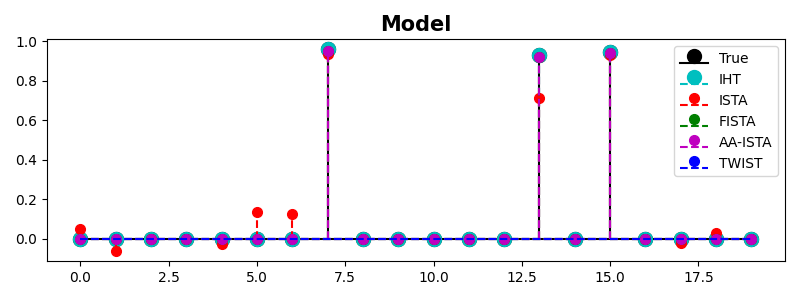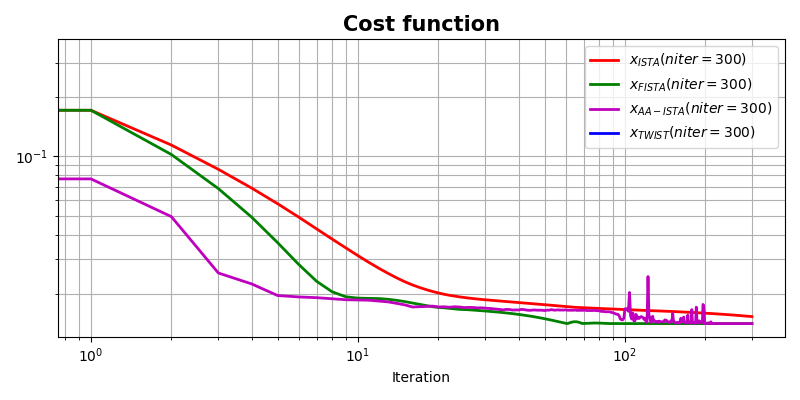# ISTA, FISTA, and TWIST for Compressive sensing#

In this example we want to compare three popular solvers in compressive sensing problem, namely pylops.optimization.sparsity.ista, pylops.optimization.sparsity.fista, and pyproximal.optimization.primal.TwIST.

Whilst all solvers try to solve an unconstrained problem with a L1 regularization term:

$J = \|\mathbf{d} - \mathbf{Op} \mathbf{x}\|_2 + \epsilon \|\mathbf{x}\|_1$

their convergence speed is different, which is something we want to focus in this tutorial.

import numpy as np
import matplotlib.pyplot as plt

import pylops
import pyproximal

plt.close('all')
np.random.seed(0)


Let’s start by creating a dense mixing matrix and a sparse signal. Note that the mixing matrix leads to an underdetermined system of equations ($$N < M$$) so being able to add some extra prior information regarding the sparsity of our desired model is essential to be able to invert such a system.

N, M = 15, 20
A = np.random.randn(N, M)
A = A / np.linalg.norm(A, axis=0)
Aop = pylops.MatrixMult(A)
Aop.explicit = False  # temporary solution whilst PyLops gets updated

x = np.random.rand(M)
x[x < 0.9] = 0
y = Aop * x


We try now to recover the sparse signal with our 3 different solvers

eps = 1e-2
maxit = 100

# ISTA
x_ista, niteri, costi = \
pylops.optimization.sparsity.ista(Aop, y, niter=maxit, eps=eps, tol=1e-10,
show=False)

# FISTA
x_fista, niterf, costf = \
pylops.optimization.sparsity.fista(Aop, y, niter=maxit, eps=eps,
tol=1e-10, show=False)

# TWIST (Note that since the smallest eigenvalue is zero, we arbitrarily
# choose a small value for the solver to converge stably)
l1 = pyproximal.proximal.L1(sigma=eps)
eigs = (Aop.H * Aop).eigs()
eigs = (np.abs(eigs), 5e-1)
x_twist, costt = \
pyproximal.optimization.primal.TwIST(l1, Aop, y, eigs=eigs,
x0=np.zeros(M), niter=maxit,
show=False, returncost=True)

fig, ax = plt.subplots(1, 1, figsize=(8, 3))
m, s, b = ax.stem(x, linefmt='k', basefmt='k',
markerfmt='ko', label='True')
plt.setp(m, markersize=10)
m, s, b = ax.stem(x_ista, linefmt='--r', basefmt='--r',
markerfmt='ro', label='ISTA')
plt.setp(m, markersize=7)
m, s, b = ax.stem(x_fista, linefmt='--g', basefmt='--g',
markerfmt='go', label='FISTA')
plt.setp(m, markersize=7)
m, s, b = ax.stem(x_twist, linefmt='--b', basefmt='--b',
markerfmt='bo', label='TWIST')
plt.setp(m, markersize=7)
ax.set_title('Model', size=15, fontweight='bold')
ax.legend()
plt.tight_layout()

fig, ax = plt.subplots(1, 1, figsize=(8, 3))
ax.semilogy(costi, 'r', lw=2, label=r'$x_{ISTA} (niter=%d)$' % niteri)
ax.semilogy(costf, 'g', lw=2, label=r'$x_{FISTA} (niter=%d)$' % niterf)
ax.semilogy(costt, 'b', lw=2, label=r'$x_{TWIST} (niter=%d)$' % maxit)
ax.set_title('Cost function', size=15, fontweight='bold')
ax.set_xlabel('Iteration')
ax.legend()
ax.grid(True, which='both')
plt.tight_layout()

••Total running time of the script: (0 minutes 0.702 seconds)

Gallery generated by Sphinx-Gallery